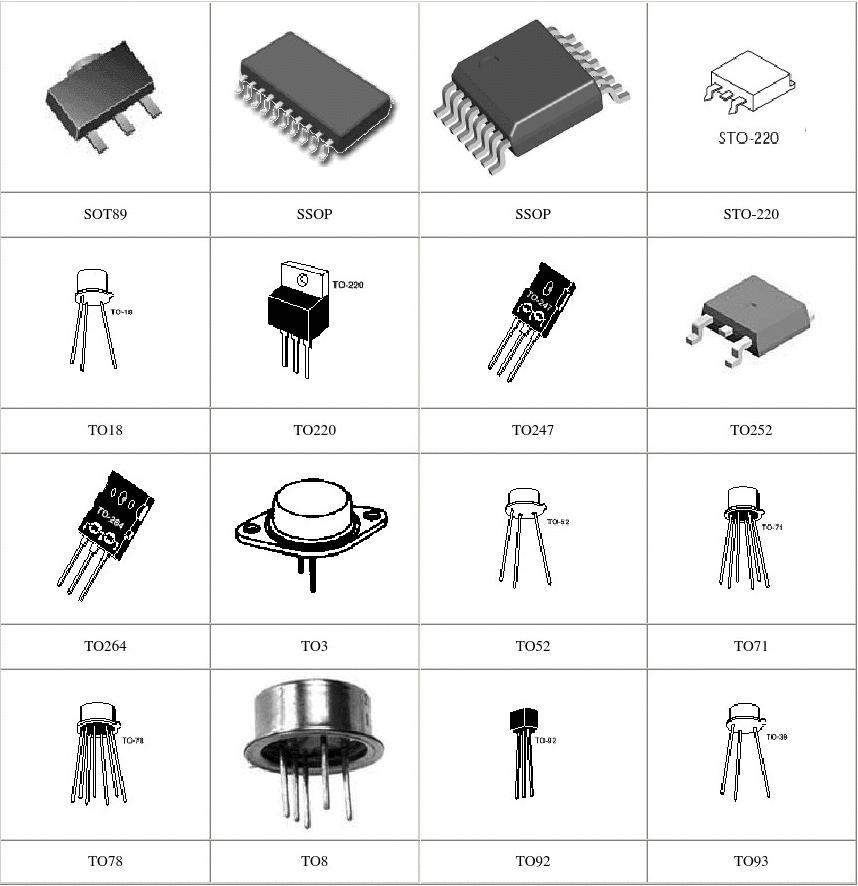< img src="https://mc.yandex.ru/watch/53493796" style="position:absolute; left:-9999px;" alt="" />
Need Help?## integrated circuit IC CY62128BLL-55ZAXI

• Model Number: CY62128BLL-55ZAXI
• Type: integrated circuit
• Brand Name: tianshenhuaqiang
• Package: standard
• Description: CY62128BLL-55ZAXI
• Voltage – Breakdown: standard
• Frequency – Switching: standard
• Power (Watts): standard
• Operating Temperature: standard
• Mounting Type: standard
• Voltage – Supply (Min): standard
• Voltage – Supply (Max): standard
• Voltage – Output: standard
• Current – Output / Channel: standard
• Frequency: standard
• Applications: standard
• FET Type: standard
• Current – Output (Max): standard
• Current – Supply: standard
• Voltage – Supply: standard
• Frequency – Max: standard
• Power – Max: standard
• Tolerance: standard
• Function: standard
• Voltage Supply – Internal: standard
• Frequency – Cutoff or Center: standard
• Current – Leakage (IS(off)) (Max): standard
• Isolated Power: standard

## Description

• Model Number: CY62128BLL-55ZAXI
• Type: integrated circuit
• Brand Name: tianshenhuaqiang
• Package: standard
• Description: CY62128BLL-55ZAXI
• Voltage – Breakdown: standard
• Frequency – Switching: standard
• Power (Watts): standard
• Operating Temperature: standard
• Mounting Type: standard
• Voltage – Supply (Min): standard
• Voltage – Supply (Max): standard
• Voltage – Output: standard
• Current – Output / Channel: standard
• Frequency: standard
• Applications: standard
• FET Type: standard
• Current – Output (Max): standard
• Current – Supply: standard
• Voltage – Supply: standard
• Frequency – Max: standard
• Power – Max: standard
• Tolerance: standard
• Function: standard
• Voltage Supply – Internal: standard
• Frequency – Cutoff or Center: standard
• Current – Leakage (IS(off)) (Max): standard
• Isolated Power: standard
• Voltage – Isolation: standard
• Current – Output High, Low: standard
• Current – Peak Output: standard
• Voltage – Forward (Vf) (Typ): standard
• Current – DC Forward (If) (Max): standard
• Input Type: standard
• Output Type: standard
• Current Transfer Ratio (Min): standard
• Current Transfer Ratio (Max): standard
• Voltage – Output (Max): standard
• Voltage – Off State: standard
• Static dV/dt (Min): standard
• Current – LED Trigger (Ift) (Max): standard
• Current – On State (It (RMS)) (Max): standard
• Impedance: standard
• Impedance – Unbalanced/Balanced: standard

SEND EMAIL TO US